# Evaluate 12

Evaluate and simplify 9 divided by 7/6.

x =  7.7143

### Step-by-step explanation: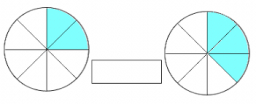Did you find an error or inaccuracy? Feel free to write us. Thank you!Tips to related online calculators
Need help to calculate sum, simplify or multiply fractions? Try our fraction calculator.
Do you want to perform natural numbers division - find the quotient and remainder?

## Related math problems and questions:

• Evaluate expressionEvaluate expression using BODMAS rule: 1 1/4+1 1/5÷3/5-5/8
• Fractions and mixed numerals(a) Convert the following mixed numbers to improper fractions. i. 3 5/8 ii. 7 7/6 (b) Convert the following improper fraction to a mixed number. i. 13/4 ii. 78/5 (c) Simplify these fractions to their lowest terms. i. 36/42 ii. 27/45 2. evaluate the follow
• Double division0.25 divided by 1/2 divided by 14
• Simplify 7Simplify. 7-1/3÷ 3-2/3 of 2+ 4-1/2÷ 2-1/4+ 1/2 solution and by step by step
• Numbers divisionWith what number should be divided mixed number 2 3/4 to get 11/12?
• Evaluate expr with 2 varsIf a=6 and x=2, find the value of (2ax + 7x- 10) /(4ax-3a-2)
• Evaluate expressionEvaluate following expression: 5 to the power of 2 - (4x4) divided by 2 =
• Two divided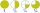Two divided by nine-tenths.
• A teacher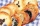A teacher baked 5 dozen cookies for the 16 students in her class. If the teacher divided the cookies equally among her students, how many cookies did she give each student?
• Evaluate mixed expressionsWhich of the following is equal to 4 and 2 over 3 divided by 3 and 1 over 2? A. 4 and 2 over 3 times 3 and 2 over 1 B. 14 over 3 times 2 over 7 C. 14 over 3 times 7 over 2 D. 42 over 3 times 2 over 31
• Evaluate expression 2Evaluate expression with negatives: (-3)+4+(-8)+(-6)+4+(-1)
• Simplify 9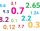Simplify and express the result as a rational number in its simplest form 1/2+ 1/5+ 6.25+0.25
• How many 17How many fifths are there in four wholes? How many 1/5 are there in 4?
• Quotient and productIf the quotient of [8/5 divided by 8/10] is added to the product of [8/14 x 7/12 x 3/8], what is the sum?
• Simplify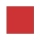Simplify powers multiplication: (3+22)(5-42)
• Simplify:Simplify: 8(−9 − 5x) + 4b use x = 9 and b = −9
• Simplify 3Simplify mixed numerals expression: 8 1/4- 3 2/5 - (2 1/3 - 1/4) Show your solution.